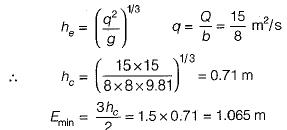Courses

# Test: Flow in Open Channel - 1

## 10 Questions MCQ Test Topicwise Question Bank for Mechanical Engineering | Test: Flow in Open Channel - 1

Description
This mock test of Test: Flow in Open Channel - 1 for Mechanical Engineering helps you for every Mechanical Engineering entrance exam. This contains 10 Multiple Choice Questions for Mechanical Engineering Test: Flow in Open Channel - 1 (mcq) to study with solutions a complete question bank. The solved questions answers in this Test: Flow in Open Channel - 1 quiz give you a good mix of easy questions and tough questions. Mechanical Engineering students definitely take this Test: Flow in Open Channel - 1 exercise for a better result in the exam. You can find other Test: Flow in Open Channel - 1 extra questions, long questions & short questions for Mechanical Engineering on EduRev as well by searching above.
QUESTION: 1

Solution:
QUESTION: 2

### in defining a Froude number applicable to channel flow, the length parameter used is

Solution: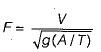where A is area and Tis top width.

QUESTION: 3

### Froude number is a significant parameter in the study of

Solution:
QUESTION: 4

Match List-1 (Flow) with List-II (Value of Froude number) the following: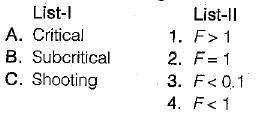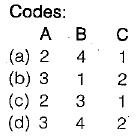Solution:
QUESTION: 5

Subcritical flow always occurs when the depth is

Solution: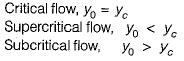QUESTION: 6

The specific energy in a channel is defined as

Solution: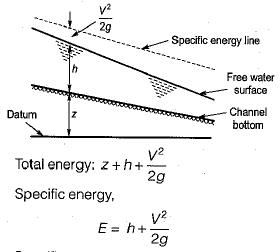Specific energy of a flowing liquid is defined as energy per unit weight of the liquid with respect to the bottom of the channel.

QUESTION: 7

At critical detph of flow

Solution:
QUESTION: 8

The critical depth hc is given by .

where q the flow rate per unit width of channel

Solution: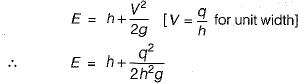for critical depth,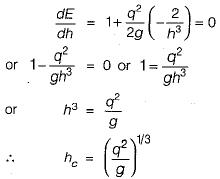QUESTION: 9

A 4 m wide rectangular channel conveys 16 m3/s of water at a depth of 2.5 m. The specific energy would be about

Solution: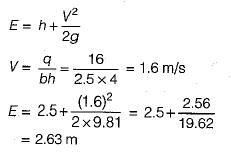QUESTION: 10

The discharge of water through a rectangular channel of width 8 m, is 15 m3/s when depth of flow of water is 1.2 m. What is value of minimum specific energy?

Solution:

Critical depth